Home  │ Audio Home Page

Updated March 21, 2017.  See Document History at end for details.

# Sealed-box Speaker Equalizer

Part 1:  Chebychev design methods allow the design of speaker equalizer

### Introduction

Perhaps you thought Chebychev filters undesirable for audio because of transient overshoot.  That is true for electronics.  However speaker design regularly uses the Chebychev filter alignment.  Indeed, it would be pointless to design a ported speaker with Bessel or Butterworth alignment.  Here Chebychev calculations are used to extend the response of sealed box speakers.  Sealed box speakers are functionally mechanical second-order filters for woofer and enclosure operation.  Therefore adding an appropriate second-order electronic stage will create a fourth-order Chebychev response with a lower cutoff frequency.

### Calculations

First calculate 4th order Butterworth poles.

 (1) θpole-i = 90° +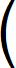180° × (2i – 1) 2N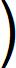(2) αi + jβi = cos(θpole-i) + jsin(θpole-i)

 Figure 1:  4th order lowpass Butterworth pole plot.Then one 4th order Chebychev pole is calculated on the unit circle from the speaker Q.

 (3) α2'' = –1 2Qspkr

 (4) β2'' = 1 – α2'2

Then that pole is scaled so that the imaginary part has the same value as the corresponding 2nd Butterworth pole.

 (5) α2' + jβ2' = (α2'' + jβ2'') × α2 α2''

 Figure 2:  Typical 4th order lowpass Chebychev pole plot.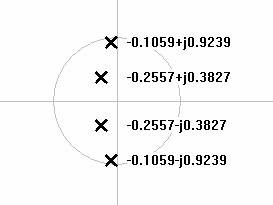Now the ratio of the real part of the Chebychev pole to the real part of the Butterworth pole gives the Chebychev constant.

 (6) kcheby = α2' α2

kcheby is all that is necessary to design the filter, however it is desirable to calculate the resulting 4th order design parameters at this time.

 (7) A = tan-1(kcheby)

 (8) ε = 1 sinh(A × n)

 (9) rippledB = 10 × log10(ε2 + 1)

 (10) f3dB = fspkr × α2'2 + β2'2

 (11) fripple = f3dB × cosh(A)

Now using kcheby calculate first Chebychev pole representing the filter.

 (12) α1' + jβ1' = kcheb⋅α1 + jβ1

Then calculate filter Q and resonant frequency.

 (13) Qfilter = 1 2cosθ = α1'2 + β1'2 2α1'

(14)
ffilter =
f3dB

 α1'2 + β1'2

### Program

Because this article pertained to an actual application, I decided to present a program rather than just an example.  The program of figure 4 calculates the filter, shows its specifications, then prints the SPICE model for it.  The resulting SPICE model represents the topology show below in figure 3.and is verified to run on SPICE Opus.  I chose C++ for the program rather than C because I wanted to use complex numbers.

 Figure 3:  Unity gain VCVS filter topology chosen for program.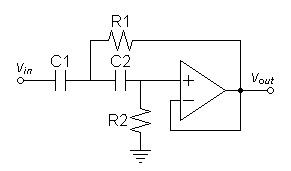Figure 4:  Code listing for filter program sbeq.cpp: #include #include #include #include // #include //include inverse hyperbolic functions. using namespace std; #define ORDER 4 #define sqr(x) ((x)*(x)) int main(int argc, char* argv[]) {     int p, ret;     double qmin,f,q,f2,f3db,fripple,q2,thetap,pi,kcheby,A,epsilon,dbripple;     double fsf, c1c2, c1c2u, r1, r2;     complex buttp, chebp;     pi = acos(-1);        // calculate first two poles of 4th order Butterworth     for(p=0;p<2;p++)     {         thetap = (pi/2.0)+(2.0*(p+1)-1.0)*pi/(2.0*(double)ORDER);         // cout << thetap*180.0/pi << endl;         buttp[p] = complex(cos(thetap),sin(thetap));     }     qmin = abs(buttp)/(2.0*fabs(real(buttp)));     // get f and q from command line.     ret = 0;     if(argc == 4)     {         ret = sscanf(argv,"%lg",&f);         if(ret)             ret = sscanf(argv,"%lg",&q);         if(ret)             ret = sscanf(argv,"%lg",&c1c2u);     }     // else get them from input.     do     {         if(!ret)         {             cout << "Enter frequency, Q, and C1/C2 value in microfarads" << endl;             cin >> f >> q >> c1c2u;         }         if(q <= qmin)         {             cout << "Quality factor must be greater than " << qmin << endl;             // prevent command line operation from getting stuck             if(argc == 3)                 return -1;         }     } // loop back until q is correct     while(q <= qmin);     // Calculate existing Chebychev pole     chebp = complex(-1.0/(q*2.0),sqrt(1.0-sqr(-1.0/(q*2.0))));     chebp *= complex(imag(buttp)/imag(chebp));     // Calculate design constants     kcheby = real(chebp)/real(buttp);     A = atanh(kcheby);     epsilon = 1.0/sinh(A*ORDER);     dbripple = 10.0*log10(sqr(epsilon)+1.0);     f3db = f*abs(chebp);     fripple = f3db*cosh(A);     // Calculate remaining Chebychev pole     chebp = complex(real(buttp * kcheby),imag(buttp));     q2 = fabs(0.5/cos(arg(chebp)));     f2 = f3db/abs(chebp);     for(p = 0; p < 2; p++)         cout << "buttp[" << p << "] = (" << real(buttp[p]) << "," << imag(buttp[p]) << ")" << endl;     cout << endl;     for(p = 0; p < 2; p++)         cout << "chebp[" << p << "] = (" << real(chebp[p]) << "," << imag(chebp[p]) << ")" << endl;     cout << endl;     cout << "Filter frequency = " << f2 << endl;     cout << "Filter Q = "   << q2 << endl;     cout << "System dB ripple = " << dbripple << endl;     cout << "System -3dB frequency = " << f3db << endl;     cout << "System ripple frequency = " << fripple << endl << endl;     // calculate unity gain Sallen and Key hardware.     c1c2 = c1c2u * 1.0e-6;     fsf = 1.0/(2*pi*f3db*c1c2);     for(p = 0; p < 2; p++)     {         r1[p] = -real(chebp[p])*fsf;         r2[p] = -norm(chebp[p])/real(chebp[p])*fsf;     }     // output SPICE model     cout <<    "* filter simulation for input parameters f = " << f << "Hz and q = " << q <

Note that if you compile the program in Visual C++ 6.0 you will have to include your own library of inverse hyperbolic functions.  Linux has them already and other compilers perhaps as well.

To compile in Linux enter:
g++ <c++ filename> -lm -o <executable filename>

If you named the program sbeq.cpp enter:
g++ sbeq.cpp -lm -o sbeq

Make the program executable:
chmod +x sbeq

Then run it:
./sbeq

Program input may be done during execution, however if you want the output to go to a file (to get the SPICE model) enter the parameters on the command line:
./sbeq <spkr resonant freq> <spkr Q> <C1/C2 value in microfarads> > <output file>
i.e.
./sbeq 50.0 0.9 0.1 > sbeq.txt

 Figure 5:  Program output for for input parameters f = 45Hz and q = 0.9 not showing SPICE model. buttp = (-0.382683,0.92388) buttp = (-0.92388,0.382683) chebp = (-0.105911,0.92388) chebp = (-0.255691,0.382683) Filter frequency = 22.2715 Filter Q = 4.39016 System dB ripple = 1.79509 System -3dB frequency = 20.711 System ripple frequency = 21.5528

 Figure 6:  Frequency response for speaker and filter for input parameters f = 45Hz and q = 0.9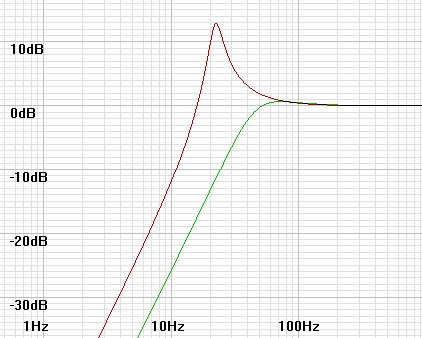Figure 7:  Combined frequency response for input parameters f = 45Hz and q = 0.9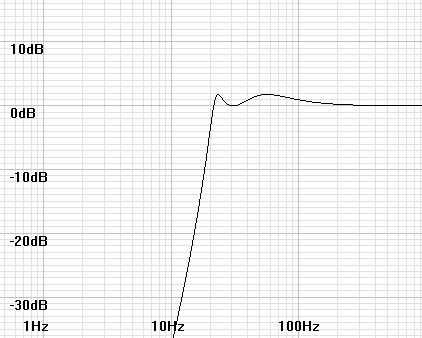### SPICE Simulation

Verify your design by running the SPICE model generated by the program.  Before doing so delete or comment out the non-SPICE lines.

 Figure 8:  SPICE model generated by program for input parameters f = 45Hz and q = 0.9 * filter simulation for input parameters f = 45Hz and q = 0.9 v1 vin 0 dc 0 ac 1 sin 0 1V 1kHz rs vin 0 100k * filter emulating speaker response c3 vin c3c4 0.1u c4 c3c4 c4r4 0.1u r3 e2out c3c4 19648.8 r4 c4r4 0 63662 e2 e2out 0 c4r4 0 1 * electonic equalizer filter c1 e2out c1c2 0.1u c2 c1c2 c2r2 0.1u r1 e1out c1c2 8138.78 r2 c2r2 0 627452 e1 e1out 0 c2r2 0 1 * rl e1out 0 100k .end .control ac dec 30 1 100 plot db(e1out) db(e2out) db(e1out/e2out) .endc

 Figure 9:  SPICE plot of speaker, filter, and combined responses..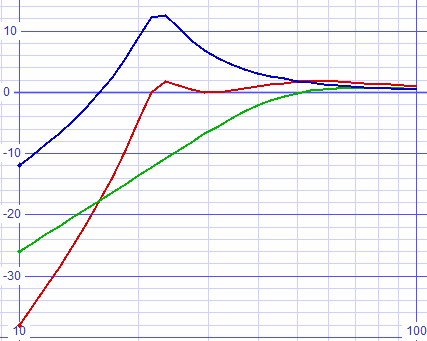Round the resistors to 1% values and reverfiy the simulation.

 Figure 10:  Same SPICE model with resistors rounded to 1% values. * filter simulation for input parameters f = 45Hz and q = 0.9 v1 vin 0 dc 0 ac 1 sin 0 1V 1kHz rs vin 0 100k * filter emulating speaker response c3 vin c3c4 0.1u c4 c3c4 c4r4 0.1u r3 e2out c3c4 19648.8 r4 c4r4 0 63662 e2 e2out 0 c4r4 0 1 * electonic equalizer filter c1 e2out c1c2 0.1u c2 c1c2 c2r2 0.1u r1 e1out c1c2 8.06k r2 c2r2 0 634k e1 e1out 0 c2r2 0 1 * rl e1out 0 100k .end .control ac dec 30 1 100 plot db(e1out) db(e2out) db(e1out/e2out) .endc

 Figure 11:  SPICE plot of speaker, filter, and combined responses, resistors rounded to 1% values..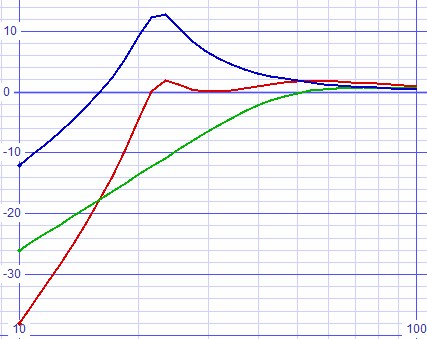### Determining speaker parameters

The difficullty in designing such a fixed filter is in knowing the required speaker specifications to some degree of precision.  If graphs of the speaker's frequency response are available the desired parameters can be obtained by inspection.  Note that away from the resonant frequency the responses approach a straight line, a horizontal one in the passband and a diagonal one in the stopband of slope 12dB/octave or 40dB/decade.  These ideal response lines are called asymptotes.  If the passband asymptote and that of the stopband are extended to where they intersect this intersection represents the resonant frequency.  Then if the actual magnitude response level at that frequency is noted then that level represented in linear terms is the same as the quality factor.  Since the graph is certain to show the magnitude in decibels rather than linear.  It is necessary to calculate the linear magnitude from the formula in equation 15 below.

 (15) Qspeaker = 10 (magnitudedB(f0) / 20)

In figure 12 below the asymptotes cross at 40Hz giving the apparent resonant frequency.  Then inspection shows the response at that frequency at ≈ –0.5.  Then calculations yield Q.

 (15) Qspeaker = 10 (–0.5dB / 20) = 0.9441

Since the magnitude is close to the asymptote away from the resonant frequency, examining the frequency where the response falls to –40dB can also help.  Then the resonant frequency is 10 times higher by knowing the asymptote slope of 40dB/decade.

 Figure 12:  2nd-order highpass plot showing how asymptotes cross at resonant frequency.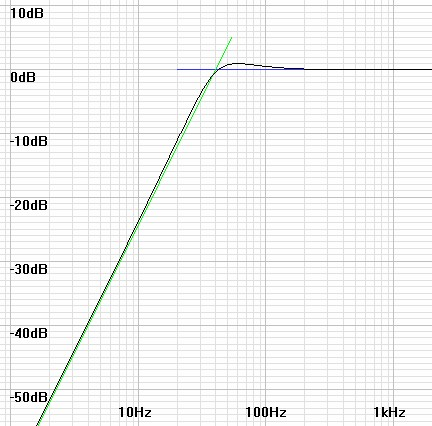### Conclusion

It is possible that other second-order highpass filter topologies could be used.  Perhaps they could be shown at another time.

1See related articles Butterworth Filter Synthesis and Chebychev Filter Synthesis.
2Hardware design calculations derived from:
Authur B. Williams and Fred J. Taylor, Electronic Filter Design Handbook, McGraw-Hill, New York, 1988.

Document History
January 14, 2017  Created.
January 14, 2017  Added missing last curly brace not copied with program and added mention of use of SPICE Opus.
January 14, 2017  Added input for user to input his own C1/C2 choice to program.
March 21, 2017  Added section Determining speaker parameters.